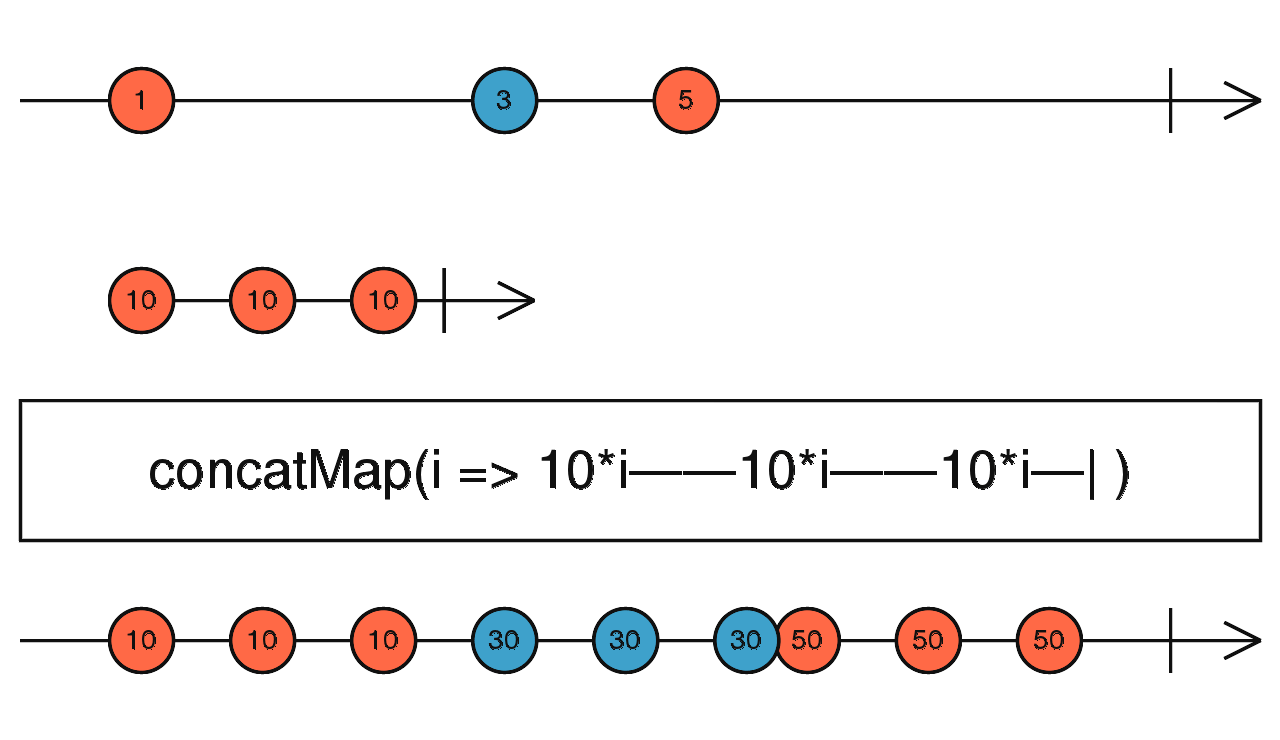/RxJS

# concatMap

`function` `stable`

Projects each source value to an Observable which is merged in the output Observable, in a serialized fashion waiting for each one to complete before merging the next.

### `concatMap<T, R, O extends ObservableInput<any>>(project: (value: T, index: number) => O, resultSelector?: (outerValue: T, innerValue: ObservedValueOf<O>, outerIndex: number, innerIndex: number) => R): OperatorFunction<T, ObservedValueOf<O> | R>`

#### Parameters

 project A function that, when applied to an item emitted by the source Observable, returns an Observable. resultSelector Optional. Default is `undefined`. Type: `(outerValue: T, innerValue: ObservedValueOf, outerIndex: number, innerIndex: number) => R`.

#### Returns

`OperatorFunction<T, ObservedValueOf<O> | R>`: An Observable that emits the result of applying the projection function (and the optional deprecated `resultSelector`) to each item emitted by the source Observable and taking values from each projected inner Observable sequentially.

## Description

Maps each value to an Observable, then flattens all of these inner Observables using `concatAll`.Returns an Observable that emits items based on applying a function that you supply to each item emitted by the source Observable, where that function returns an (so-called "inner") Observable. Each new inner Observable is concatenated with the previous inner Observable.

Warning: if source values arrive endlessly and faster than their corresponding inner Observables can complete, it will result in memory issues as inner Observables amass in an unbounded buffer waiting for their turn to be subscribed to.

Note: `concatMap` is equivalent to `mergeMap` with concurrency parameter set to `1`.

## Example

For each click event, tick every second from 0 to 3, with no concurrency

```import { fromEvent, interval } from 'rxjs';
import { concatMap, take } from 'rxjs/operators';

const clicks = fromEvent(document, 'click');
const result = clicks.pipe(
concatMap(ev => interval(1000).pipe(take(4)))
);
result.subscribe(x => console.log(x));

// Results in the following:
// (results are not concurrent)
// For every click on the "document" it will emit values 0 to 3 spaced
// on a 1000ms interval
// one click = 1000ms-> 0 -1000ms-> 1 -1000ms-> 2 -1000ms-> 3```

### `concatMap(project: (value: T, index: number) => O): OperatorFunction<T, ObservedValueOf<O>>`

#### Parameters

 project Type: `(value: T, index: number) => O`.

#### Returns

`OperatorFunction<T, ObservedValueOf<O>>`

### `concatMap(project: (value: T, index: number) => O, resultSelector: undefined): OperatorFunction<T, ObservedValueOf<O>>`

#### Parameters

 project Type: `(value: T, index: number) => O`. resultSelector Type: `undefined`.

#### Returns

`OperatorFunction<T, ObservedValueOf<O>>`

### `concatMap(project: (value: T, index: number) => O, resultSelector: (outerValue: T, innerValue: ObservedValueOf<O>, outerIndex: number, innerIndex: number) => R): OperatorFunction<T, R>`

#### Parameters

 project Type: `(value: T, index: number) => O`. resultSelector Type: `(outerValue: T, innerValue: ObservedValueOf, outerIndex: number, innerIndex: number) => R`.

#### Returns

`OperatorFunction<T, R>`